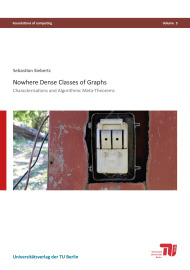# Nowhere Dense Classes of Graphs

#### Characterisations and Algorithmic Meta-TheoremsSize: 161 pages
Format: 14,8 x 21,0 cm
Publishing year: 2016
ISBN 978-3-7983-2818-1
11,00

We show that every first-order property of graphs can be decided in almost linear time on every nowhere dense class of graphs. For graph classes closed under taking subgraphs, our result is optimal (under a standard complexity theoretic assumption): it was known before that for all classes C of graphs closed under taking subgraphs, if deciding first-order properties of graphs in C is fixed-parameter tractable, parameterized by the length of the input formula, then C must be nowhere dense.
Nowhere dense graph classes form a large variety of classes of sparse graphs including the class of planar graphs, actually all classes with excluded minors, and also bounded degree graphs and graph classes of bounded expansion. For our proof, we provide two new characterisations of nowhere dense classes of graphs.
The first characterisation is in terms of a game, which explains the local structure of graphs from nowhere dense classes. The second characterisation is by the existence of sparse neighbourhood covers. On the logical side, we prove a rank-preserving version of Gaifman”s locality theorem.
The characterisation by neighbourhood covers is based on a characterisation of nowhere dense classes by generalised colouring numbers. We show several new bounds for the generalised colouring numbers on restricted graph classes, such as for proper minor closed classes and for planar graphs.
Finally, we study the parameterized complexity of the first-order model-checking problem on structures where an ordering is available to be used in formulas. We show that first-order logic on ordered structures as well as on structures with a successor relation is essentially intractable on nearly all interesting classes. On the other hand, we show that the model-checking problem of order-invariant monadic second-order logic is tractable essentially on the same classes as plain monadic second-order logic and that the model-checking problem for successor-invariant first-order logic is tractable on planar graphs.